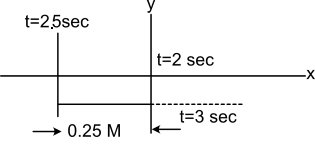Displacement in nth sec
Question

# A particle is moving along x-axis as $\mathrm{x}={\mathrm{at}}^{2}+\mathrm{bt}+\mathrm{c}$  ; where a, b and c are constant a = 1;  b = -5 and c = 6 and t is in second. Displacement of the particle during time t = 2 to t = 3 second will be

Moderate
Solution

##At t = 2 sec,  x = 0Particle will stop at t = 2.5 sec ; Distance covered by particle from t = 2 sec ; t = 2.5 second = 0.25 m ; $\therefore$  Total distance covered by particle = 0.5 m

Get Instant Solutions